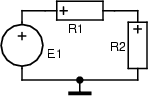Home   |  Schematics |  Products |  Tutorials  |  Datasheets  |  Robotics   |   Download    |   Link Exchange

 » Experiment » Calculator/Converters » Radio » Newsletter » Associations and Societies » Component Manufacturers

 Electronics Symentics A B C D E F G H I J K L M N O P Q R S T U V W X Y Z

 Divider Circuits Kirchhoff's Law

System

The following circuit is usually used as a voltage divider.Parameters

 rr1 = 1k [] first resistance rr2 = 2k [] second resistance

System excitation

The electrical voltage exciting the circuit is E1 = 12sin(10t).

1. Compute electrical current in the circuit, and electrical voltage across R2.

2. Using a voltage indicator test the capability of the circuit to ``divide'' electrical voltage.

3. Verify validity of the voltage Kirchhof's law in the circuit.